# Operator colligation

(diff) ← Older revision | Latest revision (diff) | Newer revision → (diff)

colligation of operators, node of operators, operator node

An aggregate of spaces and operators, to which is associated a characteristic operator-valued function. This characteristic function reflects properties of the colligation; for instance, multiplication of colligations results in multiplication of the corresponding characteristic functions. Colligations are used to build models for individual operators, but they are also employed in extension theory, interpolation theory, factorization problems, scattering theory, and system theory. They appear in different forms; the most frequently encountered forms are discussed below.

Letbe a bounded linear mapping in a Hilbert space, and assume that the imaginary part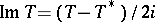ofis represented as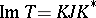, whereis a bounded linear mapping from a Hilbert spaceinto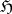and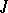is a bounded, self-adjoint and unitary operator in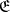(cf. also Linear operator; Self-adjoint operator; Unitary operator). The aggregate of spaces and operatorsis called an operator colligation, and the corresponding operator-valued function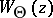acting inand defined by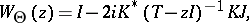is called the characteristic operator-valued function of the colligation. Clearly, the characteristic function satisfies the identitywhich exhibits many properties of. The present notion of colligation is due to M.S. Brodskii and M.S. Livšic [a6], while the characteristic function was introduced, in a slightly different form, by Livšic in [a9]. The characteristic function is a unitary invariant of the non-self-adjoint operator, and the singular points of this function coincide with the spectrum of the operator(cf. also Spectrum of an operator). The functionis a powerful tool for the investigation of the spectral properties of(invariant subspaces, triangular and functional models, Jordan representations, characterization of spectra, similarity), but it also plays a role in complex analysis (factorization into Blaschke–Potapov factors, interpolation problems); see [a3], [a17]. In system theory, the characteristic function is interpreted as the operator-valued transfer function of the system (conservative in the sense that) of the form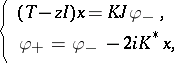where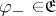is an input vector,is an output vector andis a state space vector in, so that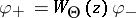. The functionis determined through the imaginary part of the non-self-adjoint operator, and therefore this function is responsible for the spectral analysis of operators "close" to bounded self-adjoint operators.

In the case where the operatoris unbounded, the Hilbert spaceis assumed to be rigged, i.e. there exists a triplet of Hilbert spaces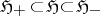(cf. also Rigged Hilbert space). Letbe a bounded linear operator from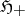intosuch that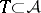and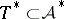. In this case, assume that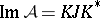, whereis a bounded linear operator from a Hilbert spaceinto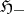andis a bounded, self-adjoint, and unitary operator in. Now the aggregate of spaces and operators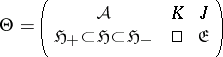is called a rigged operator colligation and the corresponding characteristic function, acting in, is defined by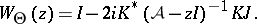In this triplet setting, the systemand(conservative in the sense that) with an input vector, an output vector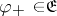and a state space vector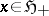, leads to. Clearly, the definition for the unbounded case preserves the algebraic formalism developed for the bounded case. Rigged operator colligations and their characteristic operator-valued functions have been introduced by E.R. Tsekanovskii and Yu.L. Shmulyan [a18]. These types of colligations (with bounded or unbounded operator) appear for instance in the theory of circuits, systems with distributed parameters and in scattering theory, see [a10], [a12]. Different definitions of characteristic functions (without colligations) for unbounded non-self-adjoint operators have been introduced and studied in [a8], [a15], [a16].

The above colligations are associated to operatorswhich are "close" to being self-adjoint. Similar notions can be developed for operators which are "close" to being unitary.

Letbe a bounded operator in a Hilbert space. Now, letand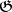be Hilbert spaces, and let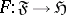,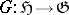andbe bounded linear operators. The aggregate of spaces and operators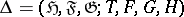is called a unitary colligation if the operator matrix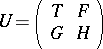is a unitary operator from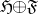onto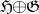. The corresponding characteristic operator-valued function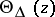, acting from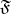to, is defined on the unit disc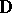by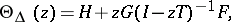whose values are contractive insideand unitary on the boundary of, as can be seen from identities such as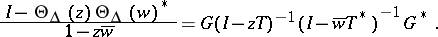The operatoris called the main operator of the colligationand it is a contraction. Conversely, any contractioninis the main operator of some unitary colligation, since the operator matrixis unitary. The spectral theory of operators "close" to unitary operators has been developed by B. Sz.-Nagy and C. Foiaş [a13] through the characteristic functions of these augmented contractions. Later the theory was reformulated in terms of unitary operator colligations and their characteristic functions, see [a4]. The colligationis called isometric, or co-isometric, if the operator matrixis isometric, respectively co-isometric. Unitary, isometric, or co-isometric colligations and their characteristic functions are being used in model theory [a19], [a14], and interpolation problems in Schur classes (cf. Schur functions in complex function theory); they also appear in extension theory, scattering theory and system theory [a2]. For instance, a discrete-time system of the form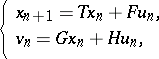with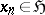,and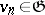,, is called conservative if the corresponding operator colligationis unitary. Solving this system by means of,and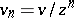, with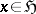,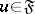,, and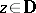, leads to.

In all types of colligations questions arise concerning the dimension of the state spaceand the minimality of the representation. Likewise, there are various constructions to build state spaces by means of characteristic functions (model theory). Operator colligations have been considered in the setting of Banach spaces [a5] and in the setting of indefinite inner product spaces (see for instance [a1] for Pontryagin spaces (cf. also Pontryagin space), and [a1], p. 205, for references for the case of Krein spaces, cf. also Krein space). Recently (1999) there is interest in colligations associated with commuting operators [a11] and in colligations with several variables [a7].

How to Cite This Entry:
Operator colligation. Encyclopedia of Mathematics. URL: http://encyclopediaofmath.org/index.php?title=Operator_colligation&oldid=12813
This article was adapted from an original article by Henk de SnooEduard Tsekanovskii (originator), which appeared in Encyclopedia of Mathematics - ISBN 1402006098. See original article